# Stokes' Theorem

Jump to: navigation, search

Stokes' Theorem states that the line integral of a closed path is equal to the surface integral of any capping surface for that path, provided that the surface normal vectors point in the same general direction as the right-hand direction for the contour:Intuitively, imagine a "capping surface" that is nearly flat with the contour. The curl is the microscopic circulation of the function on tiny loops within that surface, and their sum or integral results in canceling out all the internal circulation paths, leaving only the integration over the outer-most path. This remains true no matter how the capping surface is expanded, provided that the contour remains as its boundary.

Sometimes the circulation (the left side above) is easier to compute; other times the expresses the surface integral of the curl of vector field is easier to computer (particularly when it is zero).

Stated another way, Stokes' Theorem equates the line integral of a vector fields to a surface integral of the same vector field. For this identity to be true, the direction of the vector normal n must obey the right-hand rule for the direction of the contour, i.e., when walking along the contour the surface must be on your left.

This is an extension of Green's Theorem to surface integrals, and is also the analog in two dimensions of the Divergence Theorem. The above formulation is also called as the "Curl Theorem," to distinguish it from the more general form of the Stokes' Theorem described below.

Stokes' Theorem is useful in calculating circulation in mechanical engineering. A conservative field has a circulation (line integral on a simple, closed curve) of zero, and application of the Stokes' Theorem to such a field proves that the curl of a conservative field over the enclosed surface must also be zero.

## General Form

In its most general form, this theorem is the fundamental theorem of Exterior Calculus, and is a generalization of the Fundamental Theorem of Calculus. It states that if M is an oriented piecewise smooth manifold of dimension k and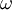is a smooth (k−1)-form with compact support on M, and ∂M denotes the boundary of M with its induced orientation, then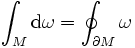,

where d is the exterior derivative.

There are a number of well-known special cases of Stokes' theorem, including one that is referred to simply as "Stokes' theorem" in less advanced treatments of mathematics, physics, and engineering:

• When k=1, and the terms appearing in the theorem are translated into their simpler form, this is just the Fundamental Theorem of Calculus.
• When k=3, this is often called Gauss' Theorem or the Divergence Theorem and is useful in vector calculus: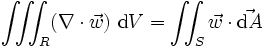Where R is some region of 3-space, S is the boundary surface of R, the triple integral denotes volume integration over R with dV as the volume element, and the double integral denotes surface integration over S with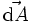as the oriented normal of the surface element. Theon the left side is the divergence operator, and theon the right side is the vector dot product.

• When k=2, this is often just called Stokes' Theorem: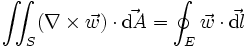Here S is a surface, E is the boundary path of S, and the single integral denotes path integration around E with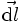as the length element. Theon the left side is the curl operator.

These last two examples (and Stokes' theorem in general) are the subject of vector calculus. They play important roles in electrodynamics. The divergence and curl operations are cornerstones of Maxwell's Equations.

Stokes' Theorem is a lower-dimension version of the Divergence Theorem, and a higher-dimension version of Green's Theorem. Green’s Theorem relates a line integral to a double integral over a region, while Stokes' Theorem relates a surface integral of the curl of a function to its line integral. Stokes' Theorem originated in 1850.